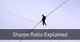# Sharpe Ratio

By Research DeskSharpe ratio calculates the excess return for an investor over the risk-free return for a certain amount of risk. It is calculated as follows:

Sharpe ratio = (Return on Portfolio - Risk Free Return) / Standard Deviation of Portfolio

where,

Return on Portfolio is the return that the investor generates on the portfolio of financial instruments which the investor is holding.

Risk Free Return is the return which can have earned by investing in zero risk instruments like a government security (g-sec).

Standard Deviation of Portfolio is the measure of the total risk of the portfolio.

Thus, a fund generating higher returns and the same standard deviation will have a higher sharpe ratio than a fund generating lower returns. It shows how much additional return can be earned by an investor by taking additional risk.

Generally, portfolio diversification with majority of assets having lower risk or no risk will reduce the standard deviation and increase the sharpe ratio.

For Example: A fund managing Rs. 10 crores invests 30% in equities having an expected return of 20%, 20% in Fixed Income Securities having a return of 15% and remaining 50% in Bonds having a return of 10%. The standard deviation of the fund is 10%. Also the return on Treasury Bonds is 8%.

In the above case, the sharpe ratio can be calculated as follows:

Portfolio Return: (20%*0.3)+(15%*0.2)+(10%*0.5) = 14%

Sharpe Ratio = (14% - 8%) / 10% = 0.6

A lower sharpe ratio indicates lower returns at a higher risk and is considered unfavourable.